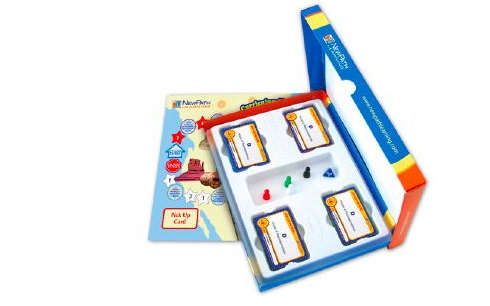# Tất cả sản phẩm# Grade 5 Math Curriculum Mastery® Game - Study-Group Edition

Mã sản phẩm: 23-5401 Lược xem: 839

Liên hệ

Includes one double-sided game board, a blended set of 250 cards representing a cross-section of the topics represented in the Class-Pack Edition (23-5001), materials for four students, and trial online subscription. Topics include:

1. Whole Numbers to Millions
2. Compare & Order Numbers
3. Place Value
4. Equivalent Fractions
5. Compare & Order Fractions
6. Order Decimals
7. Less Than, Greater Than
8. Percents as Fractions/Decimals
9. Common Factors
10. Multiply Three Digits
11. Divide Three Digits
12. Order of Operations
13. Simplify Fractions
14. Add/Subtract Fractions
15. Add/Subtract/Multiply/Divide Decimals
16. Algebra
17. Perimeter
18. Geometry Classification
19. Plot Points
20. Measurement
21. Elapsed Time
22. Angles
23. Data Analysis
24. Exponential & Scientific Notation
25. Ratios